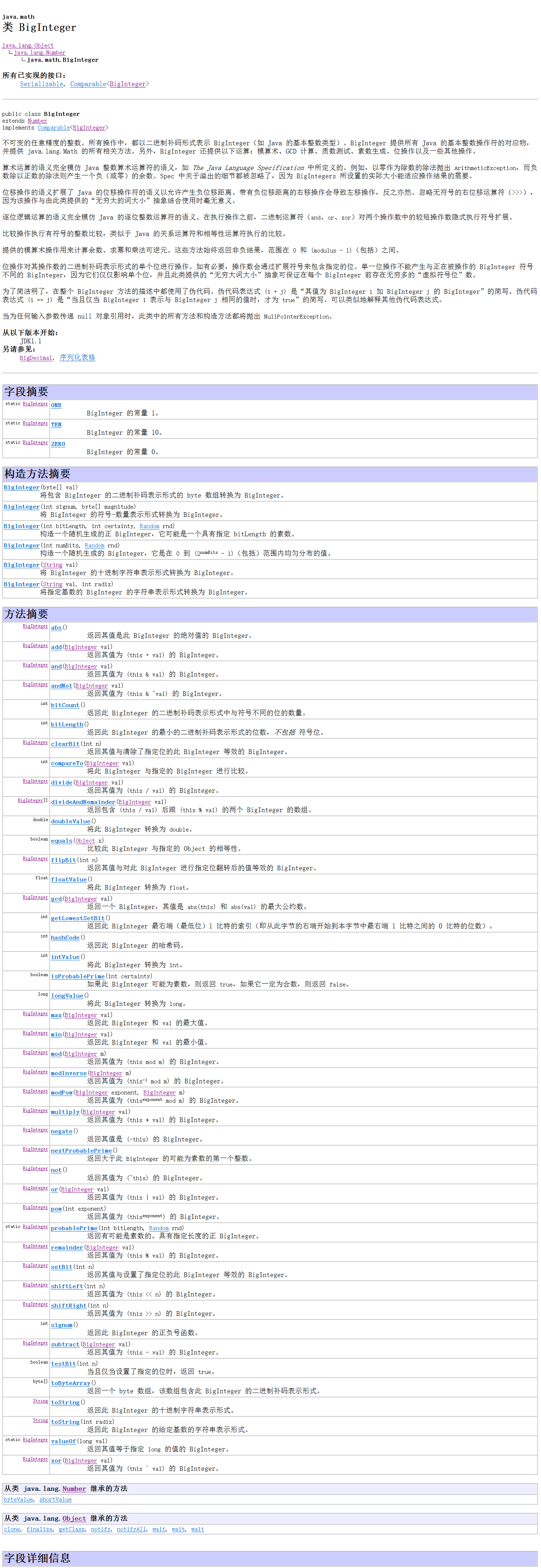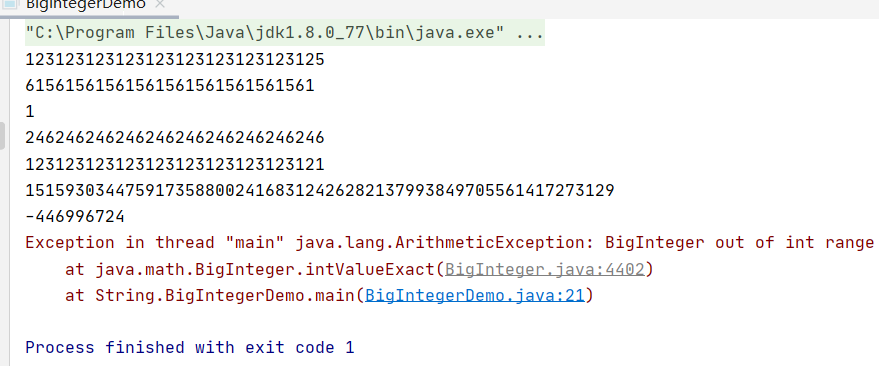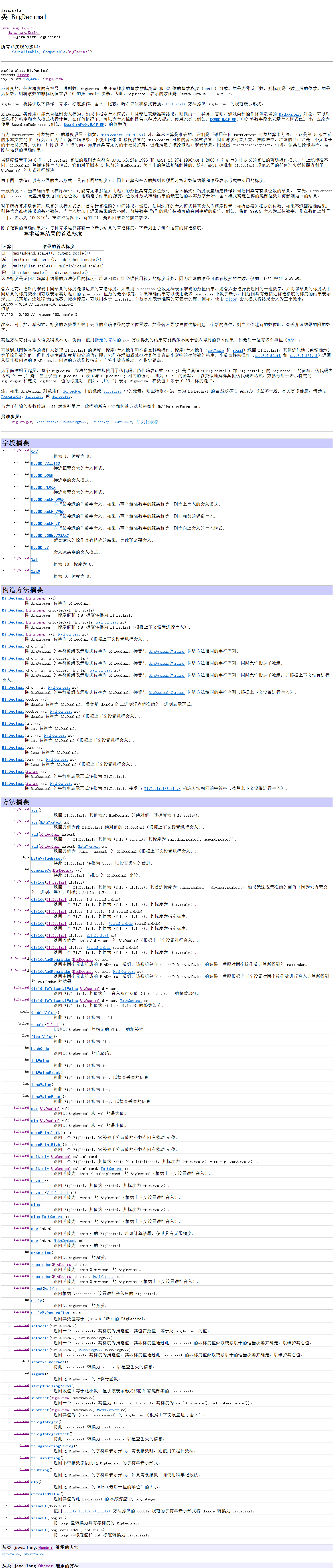BigInteger类与BigDecimal类（Java）

2021/8/21 18:04:04 浏览：

常用类解析：BigInteger类与BigDecimal类

• 前言
• 一、BigInteger类与BigDecimal类
• 二、BigInteger类
• 三、BigDecimal类

前言

Biglnteger 类和 BigDecimal 类可以用于表示任意大小和精度的整教或者十进制数。

一、BigInteger类与BigDecimal类

如果要进行非常大的数的计算或者高精度浮点值的计算，可以使用 java.math 包中的Biglnteger 类和 BigDecimal 类。它们都是不可变的。long 类型的最大整数值为 long.MAX_VALUE (即 9223372036854775807)。Biglnteger 的实例可以表示任意大小的整数。可以使用 new Biglnteger(String)和 new BigDecimal(String)来 创 建 Biglnteger 和 BigDecimal的实例，使用 add、subtract、multiple、divide 和 remainder 方法完成算术运算，使用compareTo 方法比较两个大数字。例如，下面的代码创建两个 Biglnteger 对象并且将它们进行相乘：

Biglnteger a * new BigInteger("922337203685477S807M)Biglnteger b = new BigInteger("2");
Biglnteger c = a.multiply(b); // 9223372036854775807 * 2
System.out.println(c);

对 BigDecimal 对象的精度没有限制。如果结果不能终止，那么divide 方法会抛出ArithmeticException 异常。但是，可以使用重载的 divide(BigDecimal d.int scale, int roundingMode)方法来指定尺度和舍入方式来避免这个异常，这里的 scale 是指小数点后最小的整数位数。例如，下面的代码创建两个尺度为 20、舍入方式为 BigDecimal.ROUND_UP的BigDecimal 对象。

BigDecimal a = new BigDecimal(1.0);
BigDecimal b = new BigDecimal(3):
BigDecimal c = a.divide(b, 20, BigDecimal.ROUND_UP);
System.out.println(c);

在Java中，基本数据类型中整数最长的就是 long 类型8字节 64位 -2^63 ~ 2^63 - 1，如果我们在实际应用中的数据超过了这个范围怎么办？

BigInteger专门处理大整数运算
BigDecimal专门出来大小数运算

二、BigInteger类

其实BigInteger底层就是用一个不可变的数组来维护计算我们所存储的数据的，如果通过运算得出新的数据的话 则返回新的BigInteger的对象，具体的方法参照API：在Java中，由CPU原生提供的整型最大范围是64位 long 型整数。使用 long 型整数可以直接通过CPU指令进行计算，速度非常快。
如果我们使用的整数范围超过了 long怎么办？这个时候，就只能用软件来模拟一个大整数。java.math.BigInteger 就是用来表示任意大小的整数BigInteger 内部用一个 int[] 数组来模拟一个非常大的整数：

BigInteger bi = new BigInteger("1234567890");
System.out.println(bi.pow(5)); // 2867971860299718107233761438093672048294900000

BigInteger 做运算的时候，只能使用实例方法，例如，加法运算：

BigInteger i1 = new BigInteger("1234567890");
BigInteger i2 = new BigInteger("12345678901234567890");
BigInteger sum = i1.add(i2); // 12345678902469135780

和 long 型整数运算比， BigInteger 不会有范围限制，但缺点是速度比较慢。
也可以把 BigInteger 转换成 long 型：

BigInteger i = new BigInteger("123456789000");
System.out.println(i.longValue()); // 123456789000
System.out.println(i.multiply(i).longValueExact()); // java.lang.ArithmeticException: BigInteger out of long range

使用 longValueExact() 方法时，如果超出了 long 型的范围，会抛出ArithmeticException
BigIntegerIntegerLong 一样，也是不可变类，并且也继承自 Number 类。因为Number 定义了转换为基本类型的几个方法：

• 转换为 byte ： byteValue()
• 转换为 short ： shortValue()
• 转换为 int ： intValue()
• 转换为 long ： longValue()
• 转换为 float ： floatValue()
• 转换为 double ： doubleValue()

因此，通过上述方法，可以把 BigInteger 转换成基本类型。如果 BigInteger 表示的范围超过了基本类型的范围，转换时将丢失高位信息，即结果不一定是准确的。如果需要准确地转换成基本类型，可以使用 intValueExact()longValueExact() 等方法，在转换时如果超出范围，将直接抛出 ArithmeticException 异常。
如果 BigInteger 的值甚至超过了 float 的最大范围（3.4x1038），那么返回的float是什么呢？

public class Main {
public static void main(String[] args) {
BigInteger n = new BigInteger("999999").pow(99);
float f = n.floatValue();
System.out.println(f);
}
}

BigInteger 用于表示任意大小的整数
BigInteger 是不变类，并且继承自 Number

public class BigIntegerDemo {
public static void main(String[] args) {
BigInteger b1 = new BigInteger("123123123123123123123123123123");
BigInteger b2 = new BigInteger("2");
System.out.println(b1.divide(b2));
System.out.println(b1.mod(new BigInteger("2")));
System.out.println(b1.multiply(b2));
System.out.println(b1.pow(2));

BigInteger b3 = new BigInteger("1234578459922468523401240332");
//将BigInteger对象的数字转成基本数据类型对应的数字
System.out.println(b3.intValue());//强制转换 将高位的数据截取 结果是不正确不精确

//ArithmeticException: BigInteger out of int range
System.out.println(b3.intValueExact());//温和转换 如果出现溢出截取的情况 则直接报异常 不给出计算结果
//能用带Exact用Exact的 更加安全一些
}
}三、BigDecimal类

BigDecimal底层其实是BigInteger和小数位记录scale组成的；和 BigInteger 类似， BigDecimal 可以表示一个任意大小且精度完全准确的浮点数

BigDecimal bd = new BigDecimal("123.4567");
System.out.println(bd.multiply(bd)); // 15241.55677489

BigDecimalscale() 表示小数位数，例如：

BigDecimal d1 = new BigDecimal("123.45");
BigDecimal d2 = new BigDecimal("123.4500");
BigDecimal d3 = new BigDecimal("1234500");
System.out.println(d1.scale()); // 2,两位小数
System.out.println(d2.scale()); // 4
System.out.println(d3.scale()); // 0

通过 BigDecimalstripTrailingZeros() 方法，可以将一个 BigDecimal 格式化为一个相等的，但去掉了末尾0的 BigDecimal

BigDecimal d1 = new BigDecimal("123.4500");
BigDecimal d2 = d1.stripTrailingZeros();
System.out.println(d1.scale()); // 4
System.out.println(d2.scale()); // 2,因为去掉了00

BigDecimal d3 = new BigDecimal("1234500");
BigDecimal d4 = d3.stripTrailingZeros();
System.out.println(d3.scale()); // 0
System.out.println(d4.scale()); // -2

如果一个 BigDecimalscale() 返回负数，例如， -2 ，表示这个数是个整数，并且末尾有2个0。
可以对一个 BigDecimal 设置它的 scale ，如果精度比原始值低，那么按照指定的方法进行四舍五入或者直接截断：

public class Main {
public static void main(String[] args) {
BigDecimal d1 = new BigDecimal("123.456789");
BigDecimal d2 = d1.setScale(4, RoundingMode.HALF_UP); // 四舍五入，123.4568
BigDecimal d3 = d1.setScale(4, RoundingMode.DOWN); // 直接截断，123.4567
System.out.println(d2);
System.out.println(d3);
}
}

对 BigDecimal 做加、减、乘时，精度不会丢失，但是做除法时，存在无法除尽的情况，这时，就必须指定精度以及如何进行截断：

BigDecimal d1 = new BigDecimal("123.456");
BigDecimal d2 = new BigDecimal("23.456789");
BigDecimal d3 = d1.divide(d2, 10, RoundingMode.HALF_UP); // 保留10位小数并四舍五入
BigDecimal d4 = d1.divide(d2); // 报错：ArithmeticException，因为除不尽

还可以对 BigDecimal 做除法的同时求余数：

public class Main {
public static void main(String[] args) {
BigDecimal n = new BigDecimal("12.345");
BigDecimal m = new BigDecimal("0.12");
BigDecimal[] dr = n.divideAndRemainder(m);
System.out.println(dr); // 102
System.out.println(dr); // 0.105
}
}

调用 divideAndRemainder() 方法时，返回的数组包含两个 BigDecimal ，分别是商和余数，其中商总是整数，余数不会大于除数。我们可以利用这个方法判断两个 BigDecimal 是否是整数倍数：

BigDecimal n = new BigDecimal("12.75");
BigDecimal m = new BigDecimal("0.15");
BigDecimal[] dr = n.divideAndRemainder(m);
if (dr.signum() == 0) {
// n是m的整数倍
}

在比较两个 BigDecimal 的值是否相等时，要特别注意，使用 equals() 方法不但要求两个BigDecimal 的值相等，还要求它们的 scale() 相等：

BigDecimal d1 = new BigDecimal("123.456");
BigDecimal d2 = new BigDecimal("123.45600");
System.out.println(d1.equals(d2)); // false,因为scale不同
System.out.println(d1.equals(d2.stripTrailingZeros())); // true,因为d2去除尾部0后scale变 为2
System.out.println(d1.compareTo(d2)); // 0

必须使用 compareTo() 方法来比较，它根据两个值的大小分别返回负数、正数和 0 ，分别表示小于、大于和等于。

如果查看 BigDecimal 的源码，可以发现，实际上一个 BigDecimal 是通过一个 BigInteger 和一个 scale 来表示的，即 BigInteger 表示一个完整的整数，而 scale 表示小数位数：

public class BigDecimal extends Number implements Comparable<BigDecimal> {
private final BigInteger intVal;
private final int scale;
}

BigDecimal 也是从 Number 继承的，也是不可变对象。

BigDecimal 用于表示精确的小数，常用于财务计算；

import java.math.BigDecimal;
import java.math.RoundingMode;

public class BigIntegerDemo {
public static void main(String[] args) {
BigDecimal bd1 = new BigDecimal("123.1415926");
BigDecimal bd2 = new BigDecimal("1.2700");
BigDecimal bd3 = new BigDecimal("12700");
System.out.println(bd1.multiply(bd2));
System.out.println(bd1.divide(bd2,10, RoundingMode.HALF_UP));//RoundingMode.HALF_UP：四舍五入保留(scale:)10位小数

System.out.println(bd1.scale()); //验证数字后小数的个数
System.out.println(bd2.scale());
System.out.println(bd3.scale());

System.out.println(bd2.stripTrailingZeros());
System.out.println(bd2.stripTrailingZeros().scale());

System.out.println(bd3.stripTrailingZeros());
//1.27 * 10^4
//12700
System.out.println(bd3.stripTrailingZeros().scale());//-2 最后两位是0

//无论是BigInteger该是BigDecimal 比较大小尽量不要用equals()
BigDecimal bd4 = new BigDecimal("1.2700");
BigDecimal bd5 = new BigDecimal("1.27");
System.out.println(bd4.equals(bd5));
System.out.println(bd4.compareTo(bd5));
}
}